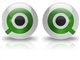# QlikView App Dev

Discussion Board for collaboration related to QlikView App Development.

Announcements
Join this live chat April 6, 10AM EST - QlikView to Qlik Sense REGISTER
cancel
Showing results for
Search instead for
Did you mean:Contributor III

## Is it possible to create a calculated value on a row?

Hi is it possible to create a calculated value on a row?

For example I have Sales, Costs1 and Costs2, is it possible to create the following:

Jan     Feb     Mar

Row n1: Sales

Row n2: Costs1

Row n3: Margin1 (Sales-Costs1)

Row n4: Costs2

Row n5: Margin2 (Margin1-Costs2)

Thanks!

Camilo Lund R.

1 Solution

Accepted SolutionsNot applicable

Remove the Group Dimension.

Create expressions

Sales:

sum({<Group={'Sales'} USD)

Cost1

sum({<Group={'Cost1'} USD)

Margin1:

sum({<Group={'Sales'} USD)-sum({<Group={'Cost1'} USD)

Cost2

sum({<Group={'Cost2'} USD)

Margin2

(sum({<Group={'Sales'} USD)-sum({<Group={'Cost1'} USD))-sum({<Group={'Cost2'} USD)

6 RepliesMVP & Luminary

Hi,

To display chart like this use Pivot table, use Month as Dimension and write expressions for Sales, Costs1, Margin1, Costs2 and Margin2.

No drag the Month dimension along to the top of the table and drag expressions to vertically.

If possible, can you attach a sample file.

Hope this helps you.

Regards,

Jagan.Contributor III
Author

Hi Jagan,

Im attaching an example hoppfully it will be more clear.

The idea would be to create a margin1 between Cost1 and Cost2 and a margin2 at the bottom.

Margin1=Sales+Cost1

Margin2=Sales+Cost1+Cost2

Thanks,

CamiloContributor III
Author

If I´m not beeing clear enough please tell me.

Thanks,

CamiloNot applicable

Remove the Group Dimension.

Create expressions

Sales:

sum({<Group={'Sales'} USD)

Cost1

sum({<Group={'Cost1'} USD)

Margin1:

sum({<Group={'Sales'} USD)-sum({<Group={'Cost1'} USD)

Cost2

sum({<Group={'Cost2'} USD)

Margin2

(sum({<Group={'Sales'} USD)-sum({<Group={'Cost1'} USD))-sum({<Group={'Cost2'} USD)Contributor III
Author

Thanks it worked, it seems an >} was mising on the set analysis commands but it worked!Not applicable

Oh, Yeah sorry about that. It has been a long day 😛